# Completion of Series

The Non Verbal Reasoning section of every competitive exam includes questions from the topic “Completion of Series”. This topic is considered to be quiet important and every year a good number of questions are asked from this topic.

A series is described as a sequential arrangement of figures following a certain pattern of transition from one to other.

Different types of questions covered in this chapter as follows

1. Choosing the Next Term in the Series

2. Choosing the Missing Figure in the Series

3. Detection of Incorrect Order in the Series

4. Detecting the Wrong Figure in the Series

LearnFrenzy provides you lots of fully solved Completion of Series Questions and Answers with explanation.

Basic Concepts of Classification - Series

Concept of Rotation

The movement of a block (figure) around a fixed point is known as rotations. The simplest example of rotation is the movement of hour and minute hand of the clock. Such movements are of two types.

1. Clockwise Rotation : When a figure rotates in the direction of the hands of a clock, then this movement is called clockwise movement. This can be better understood with the help of direction of arrows.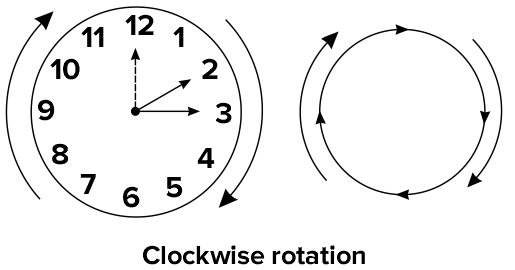2. Anti-clockwise Rotation : When a figure rotates in the opposite direction of the hands of a clock, then this movement is known as anti-clockwise movement. This can be better understood with the help of direction of arrows.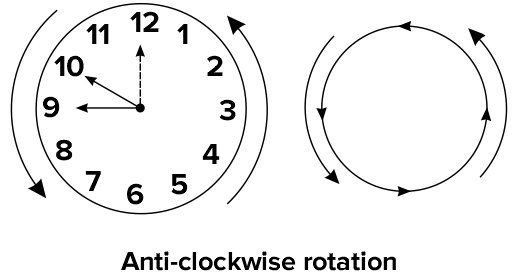Concept of Angles

The figures are rotated either clockwise or anti-clockwise by a certain angle. The concept of angles can be better understood with the help of following figure where the dotted line shows the original/initial position, dark line shows the final position and the direction arrow shows the direction of rotation.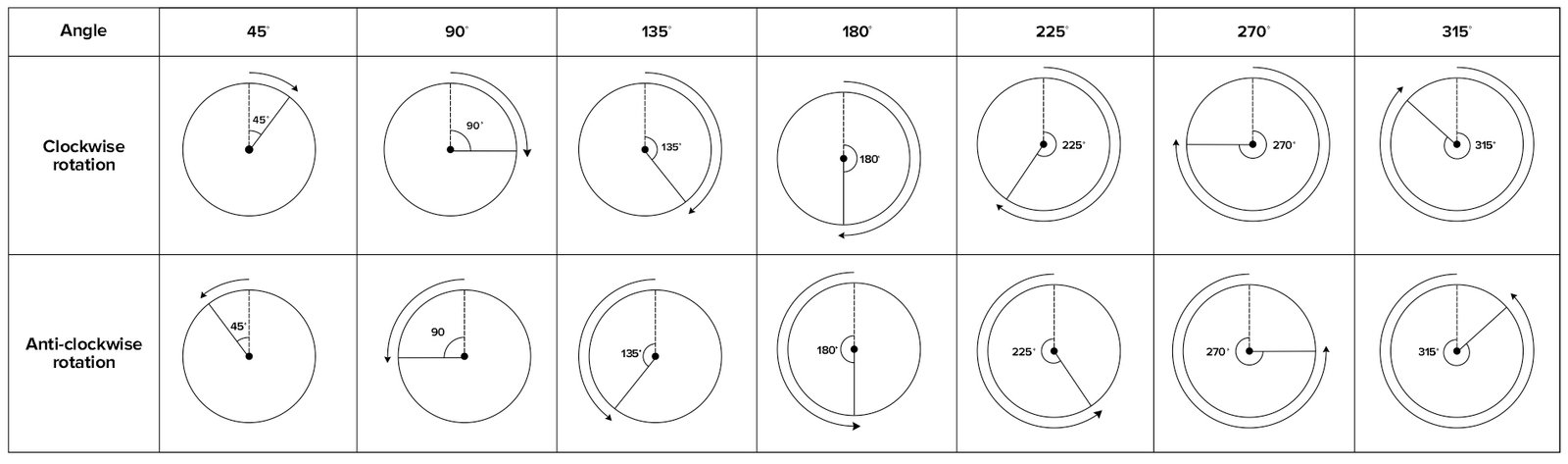Concept of Angle in a Square

The same concept of angles which is explained above is also valid for square figure as both the figures (i.e., circle and square) form an angle of 3600with the centre as shown below.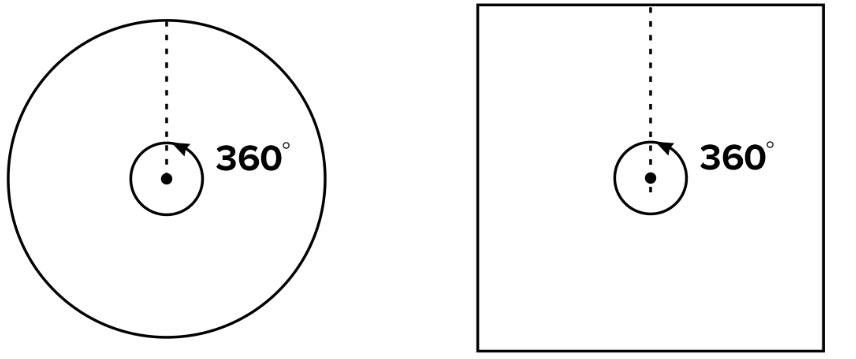Now, let us combine these two figures to have a more clear picture of the concept.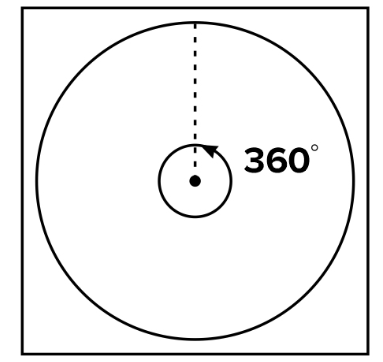So, from the above figures it is clear that the clockwise and anti-clockwise angular rotations that takes place in a square block are similar to the above explained rotations.

Concept of Steps

In some type of question based on series, the whole figure is not rotated to obtain the another figure but one or more elements/symbols inside the figure move some steps in an orderly manner to obtain the subsequent figures. So, it is required to have a clear understanding about the concept of steps.

As we have already understood the concept of angles, we can extend the concept of angles to explain the concept of steps.

Let us consider the following two figures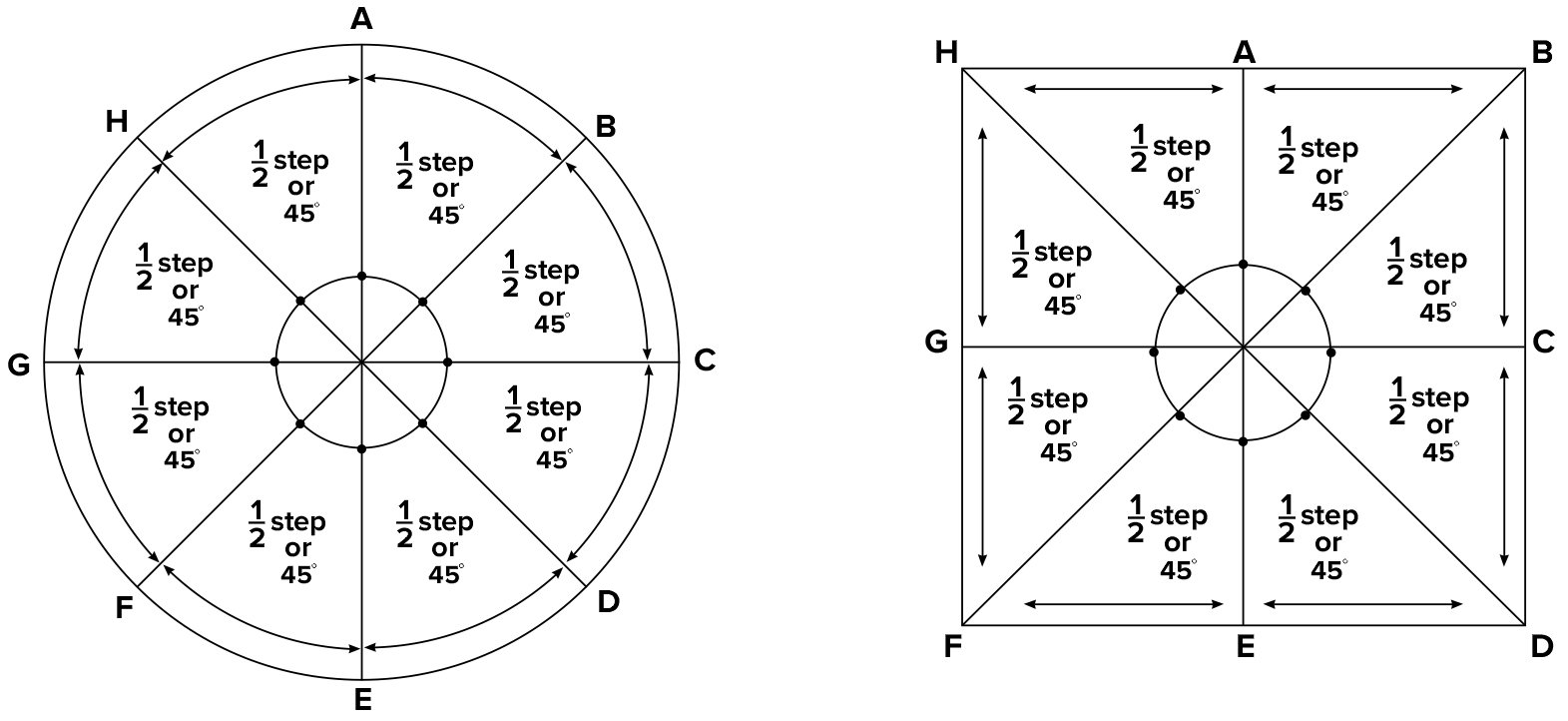From the above figure it is very much clear that the length of arc or side corresponding to 450 is taken as half step. So,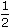step = 450